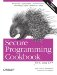# Converting a String to Lower- or Uppercase

Problem

You have a string that you want to convert to lower- or uppercase.

Solution

Use the toupper and tolower functions in the header to convert characters to upper- or lowercase. Example 4-20 shows how to do it using these functions. See the discussion for an alternative.

Example 4-20. Converting a string's case

```#include
#include
#include
#include
#include

using namespace std;

void toUpper(basic_string& s) {
for (basic_string::iterator p = s.begin( );
p != s.end( ); ++p) {
*p = toupper(*p); // toupper is for char
}
}

void toUpper(basic_string& s) {
for (basic_string::iterator p = s.begin( );
p != s.end( ); ++p) {
*p = towupper(*p); // towupper is for wchar_t
}
}

void toLower(basic_string& s) {
for (basic_string::iterator p = s.begin( );
p != s.end( ); ++p) {
*p = tolower(*p);
}
}

void toLower(basic_string& s) {
for (basic_string::iterator p = s.begin( );
p != s.end( ); ++p) {
*p = towlower(*p);
}
}

int main( ) {

string s = "shazam";
wstring ws = L"wham";

toUpper(s);
toUpper(ws);

cout << "s = " << s << endl;
wcout << "ws = " << ws << endl;

toLower(s);
toLower(ws);

cout << "s = " << s << endl;
wcout << "ws = " << ws << endl;
}```

This produces the following output:

```s = SHAZAM
ws = WHAM
s = shazam
ws = wham```

Discussion

One would think that the standard string class has a member function that converts the whole thing to upper- or lowercase, but, in fact, it doesn't. If you want to convert a string of characters to upper- or lowercase, you have to do it yourself, sort of.

Not surprisingly, there is more than one way to convert a string's case (and when I say "string," I mean a sequence of characters, either narrow or wide). The simplest way to do it is with using one of the four-character conversion functions toupper, towupper, tolower, and towlower. The first form of each of these is the narrow character version; the second form (with the extra "w") is its wide character equivalent.

Each of these functions converts the case of the character using the current global locale's rules for case conversion. Upper- and lowercase depend on the characters being used in the current locale; some characters don't have an upper- or lowercase version, in which case the functions listed above will return the same character you pass in. See Chapter 13 for more information on locales. The C++ facilities for dealing with different locales are complicated, and I cannot do them justice here.

Doing the actual character conversion is easy. Consider the toUpper function in Example 4-20:

```void toUpper(basic_string& s) {
for (basic_string::iterator p = s.begin( );
p != s.end( ); ++p) {
*p = toupper(*p);
}
}```

The line in bold does the real work. The version for wide characters is nearly identical:

```void toUpper(basic_string& s) {
for (basic_string::iterator p = s.begin( );
p != s.end( ); ++p) {
*p = towupper(*p);
}
}```

I overloaded toUpper for the different character types because there is no fully generic toupper function to convert a character's case (unless you are using facets from the header, which I discuss below). Two simple functions, as above, will get the job done.

There is another way to do this though, and the motivating factor for using this second approach would be your need to use explicit locales. The following versions of toUpper and toLower convert the case of a string, regardless of its character type, as long as the named locale (which defaults to the current locale) supports case conversion for that locale and character type.

```template
void toUpper2(basic_string& s, const locale& loc = locale( )) {
typename basic_string::iterator p;
for (p = s.begin( ); p != s.end( ); ++p) {
*p = use_facet >(loc).toupper(*p);
}
}

template
void toLower2(basic_string& s, const locale& loc = locale( )) {
typename basic_string::iterator p;
for (p = s.begin( ); p != s.end( ); ++p) {
*p = use_facet >(loc).tolower(*p);
}
}```

The lines in bold do the real work. Functionally, they work the same as the upper- and lowercase functions used in Example 4-20, except that they use the internationalization facilities in the header to do it. See Chapter 13 for a more thorough discussion of locales, facets, and internationalization.Secure Programming Cookbook for C and C++: Recipes for Cryptography, Authentication, Input Validation & More
ISBN: 0596003943
EAN: 2147483647
Year: 2006
Pages: 241